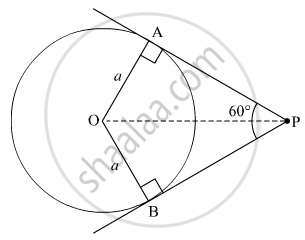Share

# If the Angle Between Two Tangents Drawn from an External Point P to a Circle of Radius a and Centre O, is 60°, Then Find the Length of Op - CBSE Class 10 - Mathematics

#### Question

If the angle between two tangents drawn from an external point P to a circle of radius a and centre O, is 60°, then find the length of OP

#### Solution

Let PA and PB be the two tangents drawn to the circle with centre O and radius a such that ∠APB=60°In ∆OPB and ∆OPA

OB = OA = a (Radii of the circle)

∠OBP = ∠OAP=90° (Tangents are perpendicular to radius at the point of contact)

BP = PA (Lengths of tangents drawn from an external point to the circle are equal)

So, ∆OPB ≌ ∆OPA (SAS Congruence Axiom)

∴ ∠OPB = ∠OPA=30° (CPCT)

Now,

In ∆OPB

sin 30^@ = "OB"/"OP"

=> 1/2 = a/(OP)

=> OP= 2a

Thus the length of OP is 2a

Is there an error in this question or solution?

#### Video TutorialsVIEW ALL 

Solution If the Angle Between Two Tangents Drawn from an External Point P to a Circle of Radius a and Centre O, is 60°, Then Find the Length of Op Concept: Tangent to a Circle.
S